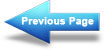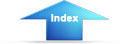Custom SearchDesign: Solar Heating of Buildings and Domestic Hot WaterMIL-HDBK-1003/13A
[tau] is transmissivity of cover glazes, [alpha] is absorptivity of collector
plate, UL is overall collector heat loss coefficient.  The y intercept
(FR([tau][alpha]n) and the slope (FRUL) can be read directly from
Table 2-6 for a wide variety of collectors.  The negative sign of the slope
is ignored and the absolute value used.  The y intercept is called efficiency
intercept in Table 2-6.  If a particular collector is not listed in Table
2-6, choose one with similar physical construction and use its value of slope
and intercept.  If necessary, slope and intercept can be calculated for a
collector shown in Duffie and Beckman (1974), but this is not recommended.
If a manufacturer's brochure is used for obtaining test data, the slope will
be a constant if the [eta] versus [delta]T/I curve is a straight line;
however, if it is not a straight line, the slope to be used is the tangent to
the curve in the expected range of [delta]T/I.  The units of FRUL must be
Btu/hr-ft2 deg. F for use on subsequent worksheets.
.
The term (mCp)c/Ac is the unit . heat capacity flowrate of working
fluid through the  collector,  where m  is flow of working fluid through the
collector in lbm/hr, and Cp is specific heat of fluid in Btu/lbm deg. F.
The larger the flowrate, the lower will be the [delta]T through collector and
thus the higher .  will be the collector efficiency.  A practical limit
is reached at  (mCp)c/Ac = 10 Btu/hr-ft2 deg. F, so the design
procedure is based on values of this order of magnitude.  The latter figure
may be taken as constant in calculating subsequent parameters.
The value for [epsilon]c, effectiveness of the collector - tank heat
exchanger, is based on manufacturer's data for the conditions of flow through
the heat exchanger.  If no heat exchanger is employed between the collector
and the tank, then this term equals 1.0.  For most heat exchangers, the
effectiveness will vary from about 0.5 to 0.8
This is a relatively complex
term to calculate and a procedure is given in U.S. Dept HUD (1977), pages
A-34 to A-39.  The simpler procedure is to ask a manufacturer for design
information.  Most manufacturers will provide this factor or calculate it.
Be prepared to supply the following information:
a.
The physical characteristics of the two fluids in the heat
exchanger.
b.
The amount of heat to be transferred (Btu/hr).
c.
The flow rates (gal/min) on both sides of the heat exchanger.
d.
The approach temperature difference defined as the difference
between the temperatures of the hot fluid entering the heat
exchanger and the heated fluid leaving the heat exchanger.
Alternatively, one could assume an effectiveness that is reasonable (say
0.7), then complete the following worksheets.  If the design appears
feasible, one could then go back and "refine" his estimate by consulting a
manufacturer to be sure that a heat exchanger is available that will provide
the assumed effectiveness.  Such a heat exchanger might be employed if the
working fluid were expensive, to reduce the amount of fluid required, or if
it were desired to separate working fluid from potable water in a hot water
storage tank (see Section 2.1.7 and Figure 2-5).
89Integrated Publishing, Inc. - A (SDVOSB) Service Disabled Veteran Owned Small Business